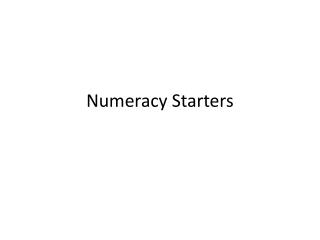DownloadDownload PresentationNumeracy Starters

# Numeracy Starters

Télécharger la présentation## Numeracy Starters

- - - - - - - - - - - - - - - - - - - - - - - - - - - E N D - - - - - - - - - - - - - - - - - - - - - - - - - - -
##### Presentation Transcript

1. Numeracy Starters

2. Put a circle around the EVEN numbers: 9 32 47 16 4 26 11 Put a circle around the ODD numbers: 16 38 21 47 33 22

3. Write in the missing numbers:a) 8 half half half b) 20 half half half

4. Write in the missing numbers:a) 8 doubled doubled doubled b) 20 doubled doubled doubled

5. You know that 3x4= 12Use it to help complete this:12÷3=12÷4=

6. Look at this number pattern53 43 33 23Write the next number in the empty box.

7. Look at this number pattern121 111 101 91Write the next number in the empty box.

8. Use these signs - + =Write the signs in the boxes to make a correct number sentence.19 13 6

9. Use these signs - + =Write the signs in the boxes to make a correct number sentence.28 12 40

10. Write the numbers in order- smallest first.31 24 50 13 42 85

11. A pencil case costs £1.65. How much will two pencil cases cost?What change will I get if I pay with a £5 note?

12. How long is this line?_______________________

13. Look at these shapes. Tick the quadrilaterals.

14. Look at these shapes. Tick the quadrilaterals.

15. Write the number that should go in the empty box.657= 600 + + 7756= + 50 + 840= 800+ +

16. Tom went into the fun play area after dinner at the time shown on the clock. What time is it?He played for 45 minutes.What time did he come out?

17. Complete this multiplication grid.

18. Complete this multiplication grid.

19. This is part of a 100 square. Fill in the missing numbers.

20. This is part of a 100 square. Fill in the missing numbers.

21. Draw all the missing linesThree tens 14Double 7 9The sum of 26 and 9 30Half of 18 35The difference between 22 and 5 17

22. Draw all the missing linesFive tens 31Double 8 14The sum of 22 and 9 50Half of 22 11The difference between 22 and 8 16

23. What fraction of the set is ring?

24. What fraction of the set is ring?

25. Colour 3/8 of this shape

26. Colour 6/12 of this shape

27. Using the information in the above box, complete the following number sentences.20 ÷ = 4 4 x = 20 ÷ 4= 5 x 4= 20

28. Using the information in the above box, complete the following number sentences.42 ÷ = 7 7 x = 42 ÷ 7= 6 x 7 = 42

29. Who am I?Show your working. Times me by 3 And subtract 7 You get 17

30. Who am I?Show your working. Times me by 3 And subtract 15 You get 25

31. Put the lottery numbers in the correct part of the Carroll Diagram. 14 9 6 38 16 35

32. Put the lottery numbers in the correct part of the Carroll Diagram. 32 5 7 35 34 68